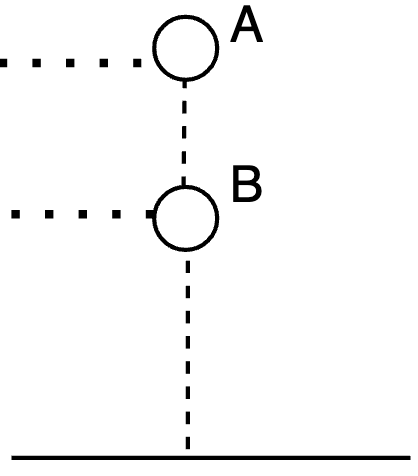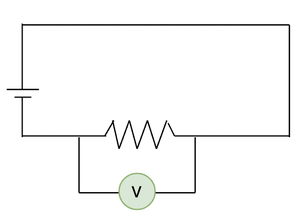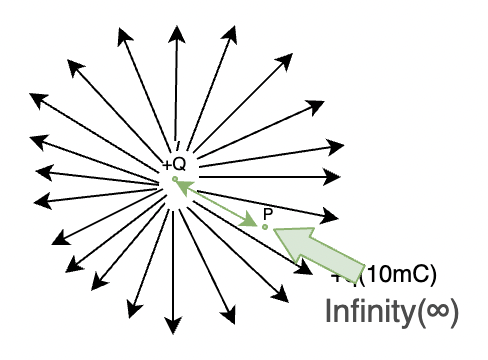Related Articles

# Electric Potential and Potential Difference

• Difficulty Level : Basic
• Last Updated : 28 Apr, 2021

The flow of Electric Charges is known as Electricity, and it is responsible for producing electric current. In this Fast Growing Era, Electricity has become really important for Human Beings, From the Fan in a small room to the heavy machines in large factories, Everything works on Electricity. An important word associated with Electricity is Electric Potential. A Potential difference is required to create the flow of electrons and hence, Produce electricity. Let’s learn about Electric potential and how a Potential difference is Created.

### Electric Potential

It is the work done per unit charge in order to bring that charge from infinity to a point in the electrostatic field against the field force. Electric Potential is also referred as Voltage. The SI unit for Electric Potential or Electric Potential difference is Voltage or Volts. Electric potential is a scalar quantity.In the above figure, +Q is the charge creating an electric field, the task is to bring a unit charge (+q) from infinity (anywhere outside the electric field) to a point inside the electric field against the field. Since, the electrostatic force produced by the electric field will be against the unit charge, some work done is required by the unit charge in order to move from either infinity to somewhere in the field, or from one point to another in the field.

• If the work is done to move the charge from infinity to point X, it will be called as Electric potential at X (Vx).
• If the work is done to move the charge from infinity to point Y, It will be called as Electric potential at Y (Vy).
• If the work is done to move the charge from X to Y, it will be called as the potential difference between x and y (Vxy).

Formula for Electric Potential:

Electric Potential/ Voltage = Work Done / Unit Charge

SI unit for Electric Potential:

V = W/q = Joules/ coulomb = Volts

Therefore, the SI unit for Electric Potential is Volts or Voltage.

1 Volt = 1 Joule/ 1 coulomb

1 Volt can be defined as 1 joule of work done in order to move 1 coulomb of charge

### Electric Potential Difference

Imagine a ball sitting at some height, will there be some energy in the ball? Yes, the energy is called as Potential energy and if the ball is dropped from a point A to B height, the ball will always fall from higher gravitational potential to lower, then there will be a difference in both the energies. The electrical potential difference is analogical to this concept.Potential Energy shown of the ball at height A and B

The energy possessed by Electric charges is known as electrical energy. A charge with higher potential will have more potential energy and the charge with lesser potential will have less potential energy. The current always moves from higher potential to lower potential. The difference in these energies per unit charge is known as the electric potential difference.

In order to create electricity and the flow of current, a potential difference is always required which is maintained by a battery or a cell.A Cell

The longer side represents Higher potential (+ve terminal) and the shorter side represents Lower potential (- terminal).

The Electric potential difference is measured by a Voltmeter which is applied parallel to the Instrument whose Voltage is to be measured.A Voltmeter

Definition of Electric potential difference

It is the work done per unit charge to move a unit charge from one point to another in an electric field. Electric potential difference is usually referred as Voltage difference.

Formula for Electric potential difference

Vxy= Vx – Vy = [Wx – Wy]/q

SI Unit of Electrical Potential Difference

The SI Unit of Electrical potential difference is same as the electric potential, i.e; Voltage or Volts.

Note: Why a unit charge is taken to explain the concept of Electric Potential?

A small unit charge will have very small electric field which will neither affect not distort the field produced by the bigger charge and hence, the concept can be explained easily.

### Ohm’s Law

According to Ohm’s Law, The current flowing through a conductor is directly proportional to the voltage provided the conductivity and temperature remains constant.

While removing the proportionality, a new constant is introduced known as the Resistance.V ∝ I

V = IR

Where, V= Voltage across the conductor

I= Current flowing in the wire

R= Resistance offered by the conductor in ohms

### Sample Problems

Question 1: A charge of 10mC is moved from infinity to a point A in Electric field, the work done in this process is 20 Joules. What is the Potential difference.

Solution:V= W/q

W= 20 joules

q= 10mC

V= 20/10×10-3= 2kV

Therefore, the potential developed is 2000volts.

Question 2: A Charge of 50mC is moved from one point to another (from A to B) The voltage at A is 50kV and the Voltage at B is 30kV, Find the Work done by the charge.

Solution:

VAB= VA– VB= 50kV- 30kV = 20kV

Work done = V× q

= 20kV × 50mC

= 100 Joules

Question 3: What are the SI units of these following quantities,

Energy, Potential Difference, Charge, Resistance

The SI Units of the above-mentioned quantities:

Energy⇢ Joules

Potential Difference⇢ Voltage

Charge⇢ Coulomb

Resistance⇢ Ohms

Question 4: Find the current through the circuit when the voltage across the terminal is 30V and the resistance offered by the conductor is 10ohm.

Solution:

According to ohms Law,

V= IR

30V = I × 10ohm

I= 3 Amperes

Therefore, the current through the circuit is 3 amperes.

Question 5: What is the difference between Electric Potential and Electric Potential difference.